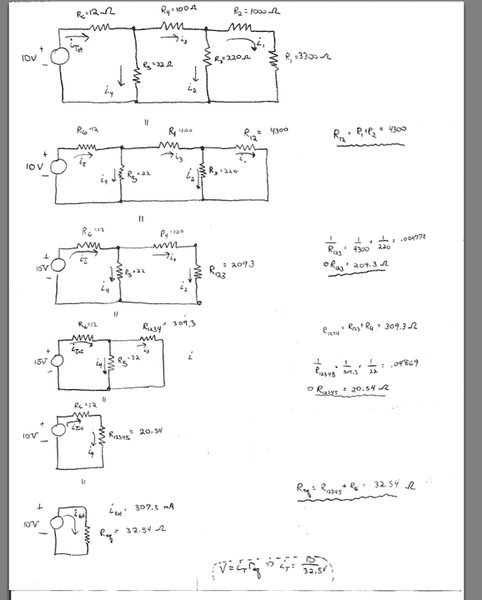## Homework Statement

I am doing a circuit lab with the voltage and resistors shown. I have already measured the currents across each resistor, but now I need to use some of the theory that we have learned in class. Here is an image of the original circuit.As you can see I have reduced it to a single equivalent circuit.

Now I am working backwards to try and find the currents shown. Now keep in mind that the index for the current i may not be the same index as its respective resistor... that is, $i_1$ does not necessarily coincide with $R_1$. I realize that that is annoying, but I did not type up the lab!

Now can someone help me to just find $i_4$ for now. I know that the current across $R_{eq}$ is 307.3 mA.

I also know that (working backwards) that $R_{eq}$ was made up from $R_{6}+R_{12345}$ which were in series with each other.

So shouldn't the current across $R_{12345}$ be the same as the i_total ? Thus making i_4=i_total=307.3 mA

Now I am pretty sure that I am not correct in saying this (because my measure currents dictate so) but I am not sure why?

Also, it says to find the currents sing the "Ladder Method"...I am assuming the above is just that.

I also have to find the currents using the "Scale Factor Method"... can someone clue me into how to do that one? I know it has to do with making an assumption and then calculating the currents based on it and this in turn leads to a scale factor.

Last edited:

Any ideas on what I am doing wrong?

farso
The way i have always done it is through Kirchoffs law.. using I1=I2+I3 then replacing the terms with ohms law V=IR and in the end getting a load nice simultanius equations, one for each loop of the circuit, in your case three.

I you don't know this method, Ill explain more if needed?

The last diagram, why have you taken out the resistor?

Last edited: